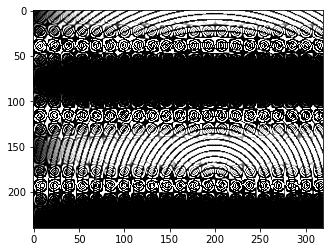Search
•1/4" Jack Of All Trades

# Creative Coding Week 5 - Drawing With Numpy

Updated: Mar 12, 2021

This weeks exercise was to attempt to create some of the plots we had done with the Mimic platform and Java in the previous term. Find some code snippets and some of the Numpy and Matplotlib drawings that I found interesting, plus basic shaded 3d shapes:

```imageWidth = 320
imageHeight = 240
center = imageWidth / 2
size = 70

myImage = np.zeros((imageHeight,imageWidth))
PI = 3.14159
width_frequency = PI / 640
frequency = width_frequency * 5000

for i in range(imageHeight):

for j in range(imageWidth):

t = math.tan(i/ frequency) * math.cos(j / frequency*(i/.1)) + math.atan(j / frequency*0.69) * math.cos(i / frequency*33)
x_dist = abs(center-i)
y_dist = abs(center-j)
dist = math.sqrt(x_dist * x_dist + y_dist * y_dist)

if math.sin(dist) < math.cos(size):
myImage[i,j] = t

plt.imshow(myImage, clim=(0,1),cmap="gray")
plt.imshow(myImage, interpolation="bilinear", clim=(0,1),cmap="gray")
plt.show()```3D Plot Code:

```from mpl_toolkits.mplot3d import Axes3D
import matplotlib.pyplot as plt
import numpy as np
from itertools import product, combinations

fig = plt.figure()
ax = fig.gca(projection='3d')

u, v = np.mgrid[0:2*np.pi:200j, 0:np.pi:100j]
x = np.cos(u)*np.sin(v)
y = np.sin(u)*np.sin(v)
z = np.tan(v)*np.cos(v)

ax.plot_surface(x, y, z, color="g")

plt.show()```